#### Theory of AC Fundamental Principles and Phasors

The Generation of AC Electricity:

When a conductor is moving in a magnetic field, an emf is induced in the conductor that has the ability to cause current to flow. Whenever a conducting loop, such as which is shown in figure below, is rotated in a magnetic field the induced emf can be evaluated between the ends of the conducting loop as a potential difference. When a means is given of making electrical contact with the ends of rotating conducting loop, then the emf can be used to supply current to a load connected between the contacts. The magnitude of the emf induced based on the strength of magnetic field, the length of the conductor forming loop and the speed at which it is rotated. It as well depends, though, on the angle at which the conducting loop moves with respect to the direction of magnetic field. This can be shown that the induced emf is proportional to sine of this angle. The emf is highest whenever the conductor is moving at right angles to the direction of magnetic field and a minimum whenever it is moving in the direction of field.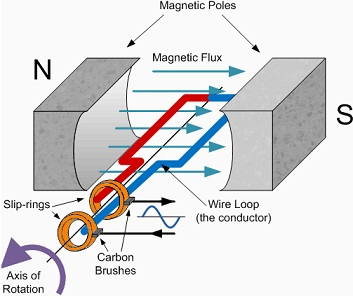Figure: A conducting Loop Rotating in a Magnetic Field

Consider the scenario where magnetic field strength is steady, as for illustration would be established by the permanent magnets. The conducting loop is of a fixed geometry and hence the length of the conductor in the magnetic field is constant. When the loop is rotated at a constant speed, then the circular angle, θ as shown in figure below, swept out by it per unit time changes at a constant rate, generally specified in radians per second. This signifies that the angle between the direction of motion of the loop and magnetic field as well changes at a constant rate.

If it is considered to begin at zero, it will increases to 90O as the loop rotates via its first quadrant and then reduces back to zero (that is, total angle relative to zero of 180O) as the loop rotates via its second quadrant. As it rotates via the third quadrant the angle will increase to 90O again, however with the arms of the loop placed in the opposite direction in the magnetic field, the polarity of induced emf is reversed. Ultimately, as the loop is rotated via the fourth quadrant the angle reduces back to zero (that is, total of 360O) again. As all the parameters of this system are constant comprising the speed of rotation, then only the angle between the direction of magnetic field and the direction of motion of conducting loop is modifying in the cyclical way explained above. The outcome is that the emf produced between the ends of the conducting loop differs in a cyclical way as the sine of this angle. This signifies that the output voltage produced will be a sinusoidal function of time as shown in figure below: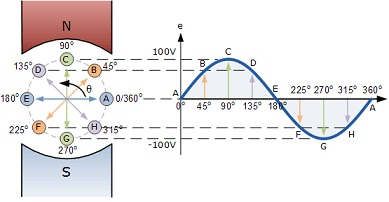Figure: Generation of an Alternating Output Voltage from Conducting Loop.

In practice various mechanical means is employed to rotate the conducting loop. This could be the crankshaft of petrol engine in a portable generator, the core of propeller of a wind turbine or the rotor driven by a water turbine positioned in the flow of a river, as for illustration at Ardnacrusha on the Shannon. These all generate circular motion of the conducting loop and hence it rotates in the magnetic field. This signifies that the vast majority of ac electric generators generate the sinusoidal type of voltage waveform as shown in figure above.

Phasors:

A phasor is a rotating vector which has fixed magnitude however phase that varies with time is as shown below. The voltage generated in the generation of ac electricity as explained above and can be symbolized by a phasor. If the phasor rotates at a steady speed or frequency then the projection of its magnitude and phase on an x-y plane outcomes in the sinusoidal profile or waveform as shown below. The horizontal x-direction might stand for phase or time and the vertical y-direction symbolizes magnitude as shown in figure below: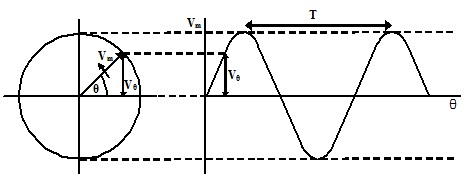Figure: The Projection of a Rotating Vector on a Vertical Axis.

The output voltage of the rotating conducting loop can then be described as:

Vθ = Vm sin θ

Where θ is the phase angle and Vm is the magnitude (exhibited as the length) of vector. The number of characteristic properties of sinusoidal function is of interest:

Angular Frequency, ω: is the rate at which the phasor modifies its rotational phase evaluated in radians per second (or degrees per second).

Cyclic Frequency, f: is the rate at which the phasor rotates in terms of number of complete cycles of 3600 it makes per second, evaluated in cycles per second or Hz.

1 cycle per second = 360 degrees per second = 2π radians per second

Then:

Vθ = Vm sin θ = Vm Sin ω = Vm sin 2Πft

And ω = 2 Πf

Period, T: One full cycle of wave occupies a time T recognized as the period of the waveform. When the frequency of sine wave is f then f full cycles take place in one second and hence the period of one cycle is given as: t

T = 1/f or f = 1/T

Peak Voltage, Vm
: This is the amplitude of sine wave or the maximum voltage achieved over one full cycle of the waveform. Note that the sinusoidal voltage waveform has a positive and negative peak which is equivalent and opposite in voltage.

Peak-to-Peak Voltage, Vptp: This is the total voltage excursion of waveform among its positive and its negative peak. In case of a sinusoidal waveform this can be seen that:

Vptp = 2Vm

Average Value, VAVE: Whenever determining the power or energy consumed by an electric circuit we are seldom fascinated in this on an instantaneous basis, apart from for analytical aims. We are most interested in the longer term or average power consumption over the prolonged period. This would recommend that the average value of sinusoidal voltage waveform is of interest. Though, it is apparent that as the sinusoidal waveform is symmetrical regarding zero, having equivalent positive and negative excursions of similar profile then the average value is zero. Though this can be officially proven: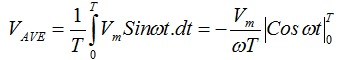VAVE = - (Vm/ ωT) (cos ωT – cos 0)

Although ω = 2 ω f    and   f = 1/T and hence ω = 2π/T or ωT = 2π and hence:

VAVE = - (Vm/2 π) (cos 2π – cos 0) = - (Vm/2 π) (1-1) = 0

This confirms that the average value of sinusoidal voltage waveform is zero. This outcome might wrongly be taken to imply that the power related with a sinusoidal voltage source is zero. Though, we are well conscious that the domestic electricity supply consumes power, even whenever joined to the simplest of resistive loads like a light bulb or a heating element. When it is recalled that in our analysis of dc circuits the power dissipated in a resistive load, R, is provided as P = V2/R, then it is perhaps more suitable to find out the average value of the square of voltage waveform when power consumption is of interest.

Root-Mean-Square, RMS Value, VRMS: This is the square-root of mean of the square of voltage waveform. In order to acquire this value it is first essential to obtain the square of the waveform over one full cycle, then to calculate the average or mean value of this and ultimately to take the square-root of the outcome.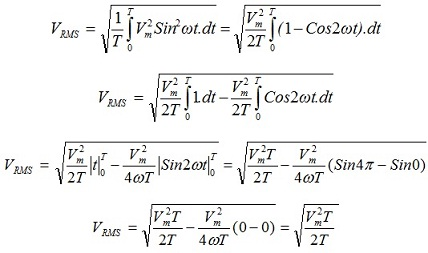And hence finally:

VRMS = Vm/√2

The rms value of sinusoidal voltage waveform is in consequence the value of an equivalent fixed dc voltage source that will dissipate similar amount of power in a load as the sinusoidal voltage does whenever averaged over a full cycle or in the long term.

Note that the value above is the value applying to the sinusoidal function only, and will be distinct for other kinds of voltage functions or waveforms.

The Complex Phase Plane:

In Engineering, and in specifically Electronic Engineering, the sinusoidal signals Sine and Cosine, that is, Sin(ωt) and Cos(ωt), are of special significance as they are both primitive harmonic functions with one being the derivative of other. In Engineering a lot analysis is concerned with rates of change and the mathematics related with this in the Calculus. As can be seen in figure below, such two signals have a 90 degrees phase shift with respect to one other.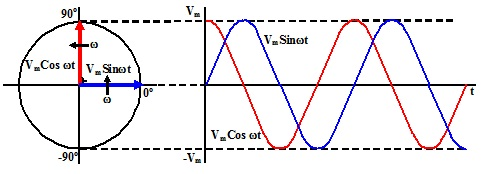Figure: Sine and Cosine Functions as Vectors

In light of this, the Argand Plane is employed as a Complex Phase Plane to symbolize components that have a ±90O phase shift with respect to one other as shown in figure below. In science and engineering mathematics the j operator (instead of i as employed in mathematics) has been established as a convenient means of symbolizing a phase shift of 90O between signals or components. This is a considerable help in the analysis of ac circuits.

j = √-1

Signal V (θ) = V (ωt)

Phase shifted by 900

V (θ + 900) = V (θ + π/2) = V (ωt + π/2)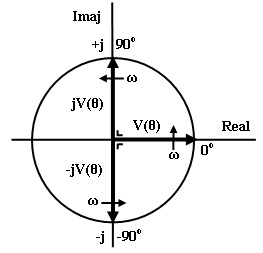Figure: Sine and Cosine Components in the Argand Plane

And hence:

V (θ + π/2) = jV(θ) = jV(ωt) and V (θ - π/2) = -jV(θ) = -jV(ωt)

However, a normally defined phasor or vector rotating in a plane can be broken into two orthogonal components that have a 90O phase shift among them that is two orthogonal components, as shown in figure below. In terms of complex plane such are termed to as the Real and Imaginary parts of the vector.

Vm = Real (Vm) + Imag (Vm

Vm = a + jb

tan θ = b/a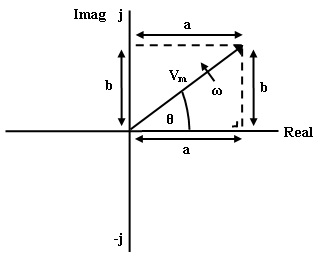Figure: A Phasor symbolized in Complex Form

Then:

a = Real (Vm) = Vm cos θ = Vm cos ωt

jb = Imag (Vm) = jVm sin θ = jVm sin ωt

By using Pythagoras theorem:

Vm2 = a2 + b2

Magnitude: |Vm| = √ a2 + b2

Phase: θm  = tan-1 b/a

In complex form, the vector is precised as:

V (t) = Vm ejωt = Vm cos ωt + jVm sin ωt

If a Vector is Vθ = a + jb then its Complex Conjugate is Vθ* = a - jb

Importance of Phasors:

The significance of phasors in Electronic Engineering is that they comprise of the most basic of electronic signals, that is, the sinusoid or sine wave. They have a very helpful property in terms of the analysis of electronic systems. When a sinusoidal signal at a given frequency and phase is represented at the input of a linear system, then the output signal (subsequent to initial transients have died away) is as well as sinusoid at similar frequency as the input signal. The output signal will encompass a different magnitude and phase than the input. The modification in magnitude and phase is in general different at different frequencies and it is this property which is termed to as the frequency response of the system. This can be evaluated in practice by using signal generators that give sine wave output signals.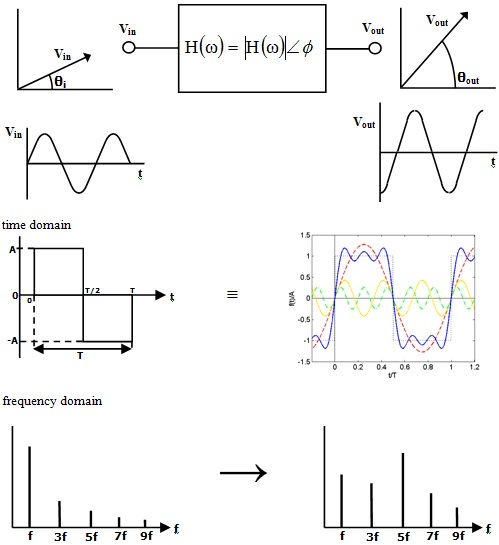Latest technology based Electrical Engineering Online Tutoring Assistance

Tutors, at the www.tutorsglobe.com, take pledge to provide full satisfaction and assurance in Electrical Engineering help via online tutoring. Students are getting 100% satisfaction by online tutors across the globe. Here you can get homework help for Electrical Engineering, project ideas and tutorials. We provide email based Electrical Engineering help. You can join us to ask queries 24x7 with live, experienced and qualified online tutors specialized in Electrical Engineering. Through Online Tutoring, you would be able to complete your homework or assignments at your home. Tutors at the TutorsGlobe are committed to provide the best quality online tutoring assistance for Electrical Engineering Homework help and assignment help services. They use their experience, as they have solved thousands of the Electrical Engineering assignments, which may help you to solve your complex issues of Electrical Engineering. TutorsGlobe assure for the best quality compliance to your homework. Compromise with quality is not in our dictionary. If we feel that we are not able to provide the homework help as per the deadline or given instruction by the student, we refund the money of the student without any delay.

2015 ©TutorsGlobe All rights reserved. TutorsGlobe Rated 4.8/5 based on 34139 reviews.